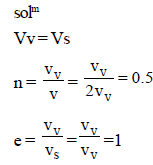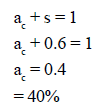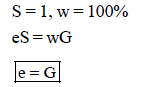Courses

# Test: Soil Mechanics - 1

## 25 Questions MCQ Test Civil Engineering SSC JE (Technical) | Test: Soil Mechanics - 1

Description
This mock test of Test: Soil Mechanics - 1 for Civil Engineering (CE) helps you for every Civil Engineering (CE) entrance exam. This contains 25 Multiple Choice Questions for Civil Engineering (CE) Test: Soil Mechanics - 1 (mcq) to study with solutions a complete question bank. The solved questions answers in this Test: Soil Mechanics - 1 quiz give you a good mix of easy questions and tough questions. Civil Engineering (CE) students definitely take this Test: Soil Mechanics - 1 exercise for a better result in the exam. You can find other Test: Soil Mechanics - 1 extra questions, long questions & short questions for Civil Engineering (CE) on EduRev as well by searching above.
QUESTION: 1

Solution:
QUESTION: 2

### Water content of soil can

Solution:

In soil Mechanics Water Content (w) = Weight of water/weight of Soil.

take 0.9 Kg of dry Soil and add water about 1 liter (in volume) which is about 1Kg in weight.

Then the water content of mixture = 1/0.9 = 1.11 = 111%

which is greater than 100%,

Mud or slurry tends to have water content more than 100%.

QUESTION: 3

### Which of the following types of soil is transported by gravitational forces?

Solution:
QUESTION: 4

A fully saturated soil is said to be

Solution:
QUESTION: 5

Total number of stress components at a point within a soil mass loaded at its boundary is

Solution:

Explanation : Considering a cube will give us 6 stresses till where it’s easy to get. And rest counting as 3 shear stresses along each plane in each direction will give total 9 stresses.

QUESTION: 6

The submerged density of soil in terms of unit weight of watergw, specific gravity G and Voids
ratio e is given by the expression

Solution:
QUESTION: 7

A soil has a bulk density of 22 kN/m3 and water content 10%. The dry density of soil is

Solution:
QUESTION: 8

If the voids of a soil mass are full of air only, the soil is termed as

Solution:
QUESTION: 9

Valid range for n, the percentage voids, is

Solution:
QUESTION: 10

Select the correct statement.

Solution:
QUESTION: 11

Voids ratio of a soil mass can

Solution:

Explanation : The void ratio is the ratio of the volume of voids (open spaces, i.e. air and water) in a soil to volume of solids. The void ratio is thus a ratio which can be greater than 1. It can also be expressed as a fraction. Void ratio and porosity differ only in the denominator.

QUESTION: 12

If the volume of voids is equal to the volume of solids in a soil mass, then the values of porosity and voids ratio respectively are

Solution:QUESTION: 13

When the degree of saturation is zero, the soil mass under consideration represents

Solution:
QUESTION: 14

In a liquid limit test, the moisture content at 10 blows was 70% and that at 100 blows was 20%. The liquid limit of the soil, is

Solution:

The liquid limit of the soil is 65 %

• Liquid limit is define as water content at which the soil possess an arbitrarily fixed small amount of shear strength.
• The result of test are represented in form of curve known as flow curve from which water content corresponding to 25 no. of blows is interpolated and is called as liquid limit.
• Graph is shown in the attached figure.

Let N be the number of blows and w be the water content at N blows.

Liquid limit = w × (N/25)⁰°¹²¹

For 10 number of blows = w (l) = 70 (10/25)⁰°¹²¹ = 89.55 %

For 100 number of blows  = 20 ( 100/25)⁰°¹²¹ = 23.65 %

So liquid limit is = 89.55 % - 23.65 % ≈ 65 %

QUESTION: 15

If the degree of saturated of a partially saturated soil is 60% then air content of the soil is

Solution:QUESTION: 16

If the water content of a fully, saturated soil mass is 100%, then the voids ratio of the sample is

Solution:QUESTION: 17

The ratio of the volume of voids to the volume of soil solids in a given soil mass, is known

Solution:
QUESTION: 18

Relative density of a compacted dense sand is approximately equal to

Solution:
QUESTION: 19

If the sand in - situ is in its densest state, then the relative density of sand is

Solution:
QUESTION: 20

Which of the following methods is most accurate for the determination of the water content of soil?

Solution:
QUESTION: 21

For proper field control which of the following methods is best suited for quick determination of water content of a soil mass?

Solution:
QUESTION: 22

A pycnometer is used to determine

Solution:
QUESTION: 23

Stoke’s law is valid only if the size of particle is

Solution:
QUESTION: 24

In hydrometer analysis for a soil mass

Solution:
QUESTION: 25

The hydrometer method of sedimentation analysis differs from the pipette analysis mainly in

Solution: